PHY294H - Lecture 44

Interference due to a thin oil film

When interference occurs due to a thin film with refractive index n, we have to take into account the fact that the wavelength of light is shorter inside the thin film. [ Recall that the speed of light inside a material with refractive index n is, vn = c/n. The frequency however is the same, so we have,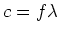and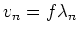. Taking the ratio of these equations yields. ] The number of wavelengths of light inside a thickness 2 l is then,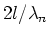. Notice that the only modification to our previous analysis is that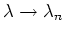.

Interferometers

We have seen that the wave nature of light leads to easily observed effects in a variety of situations, but typically when there is a length scale which is of order the wavelength of light or smaller. The typical wavelength of light is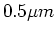, so light can be sensitive to rather small lengths. This sensitivity is used as a tool in interferometers.

Michelson interferometer

Michelson invented the interferomenter near the end of the 19th century. It was used in the famous Michelson-Morley experiment which indicated the absence of an ether''.

The Michelson interferometer produces interference fringes by splitting a beam of monochromatic light so that one beam strikes a fixed mirror (this beam has pathlength l1) and the other a movable mirror (this beam has pathlength l2. When the reflected beams are brought back together, an interference pattern results. As usual the condition for constructive interference is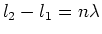. Note that if the media through which the beams travel is different for the two paths, then the path lengths l2 and l1 must be modified. For example if path l1 is through air and path l2 is through water, then the length of path 2 must be multiplied by the refractive index of water.

Fabry-Perot interferometer

The Fabry-Perot interferometer uses multiple reflections of the two beams to enhance the interference pattern. This is achieved by using two parallel mirrors one of which is a partially transmitting mirror.

Diffraction

Consider breaking up the single slit diffraction process up into 2N+1 sources equally spaced in a slit of width a. The spacing between adjacent sources is a/2N, as we place one source at each edge of the slit. The electric field at a position on the screen at angle to the center is then,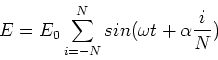(1)

where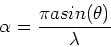(2)

Now we define x=i/N and change the sum to an integral, then(3)

Using the trigonometric identity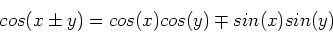(4)

we find,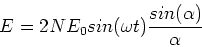(5)

The intensity is then,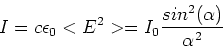(6)

Diffraction minima then occur at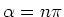where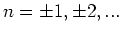or,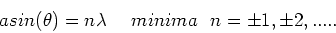(7)

In the limit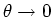, there is a diffraction maximum so that the central maximum is twice the size of all of the other maxima.

Multiple slit interference pattern

The intensity of a multiple slit interference pattern, where there are N slits separated by distance d is given by,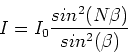(8)

where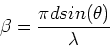(9)

This expression can be found by summing up the contributions of the site to the total electric field, as we did in the diffraction case. In fact if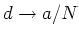with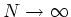, we recover the diffraction case. Principle maxima occur when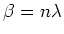or,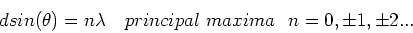(10)

which is the same as the condition for maxima in the double slit case. However the size of the principal maxima increases as N2, which implies that the angular width of these peaks is proportional to 1/N. This is very important as it allows high precision measurements of spacings by using systems with a large number of slits, i.e.. Systems with many slits are often called diffraction gratings. If we find the location of a principal maxima, and we know d to high precision, then we can find the wavelength of the light. This was a key method in finding precise values of the wavelengths of light emitted in early spectroscopic studies of atoms and molecules.